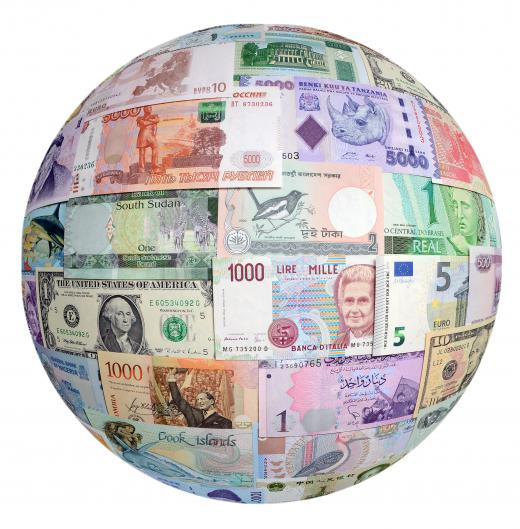# What is a Trade-Weighted Dollar?

John Lister
John ListerThe trade-weighted dollar is a way of expressing the value of the United States dollar in terms of foreign currencies.

The trade-weighted dollar is a way of expressing the value of the United States dollar (USD) in terms of foreign currencies. Rather than simply comparing it to an average of all foreign currencies, it gives added importance, or weight, to the most widely used currencies in international trade. The purpose of the trade-weighted dollar is to better measure the real USD purchasing value. A simple average of all foreign currencies would include a lot of currencies from countries where US citizens and companies do not buy many products or services. A big change in the exchange rate between currencies in such countries and the US dollar doesn't make as much difference to the overall cost of imports to Americans.With the dollar index, the euro rate is multiplied by 57.6 percent.

There are two main measures of the trade-weighted dollar. The first is the US Dollar Index, created in 1973. The second is the Trade Weighted US dollar index, sometimes known as the broad index, created in 1998. The latter was designed to more accurately reflect the countries the US trades with in the modern era. It covers 26 currencies, rather than just six in the US Dollar Index.

Both indices are weighted, which means that the figures for each exchange rate are adjusted before the average is calculated. For example, with the US Dollar Index, the euro rate is multiplied by 57.6%, while the Swiss franc rate is multiplied by 3.6%. This means that changes in the US dollar to Swiss franc rate have a much smaller effect on the overall index figure. The US Dollar Index uses a fixed set of weights, while the Trade Weighted US Dollar Index updates the weights once a year to reflect the current pattern on US foreign trade. This updating means the weight given to the euro exchange range is usually much lower for the Trade Weighted US Dollar Index than the US Dollar Index.

There are two variants on the Trade Weighted US Dollar Index. One, known as the nominal index, simply uses the current exchange rates, after adjusting, for the weighting. The second variation, known as the real index, adjusts for weights and then adjusts again to take account of prevailing inflation rates in each country. This is designed to produce a trade-weighted dollar that gives a more accurate picture of the real purchasing power of the US dollar and overcomes situations, such as where a US company can get more foreign currency for its dollars, but actually winds up being able to buy fewer goods or services.

## You might also Like